Wacker Art Gamma FunctionBild: "Ederseeschiffahrt - Die Reise nach Atlantis"

Gamma Function - Γ(z)

The Gamma Function - Γ(z) was developed by Leonhard Euler to generalise the factorial n! to non-integer values.

The following relation between the factorial and the gamma function with integer arguments is given: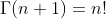Definiton

The first definition of the gamma function was given by Euler in 1730 in a letter to Goldbach.

For x > 0 we have:or in the following equivalent form: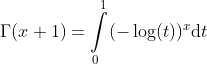By a change of variables the integral becomes the following form: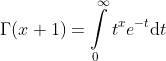Alternative Definition

The gamma function can be defined by using the following product: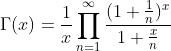Relation to the Mellin Transformation

The gamma function Γ(x) is a special case of a Mellin Transformation Mf (x):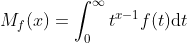The gamma function Γ(x) is the Mellin transform of f(t) = e-t.

Properties

For integer values we have:As generell formular we have: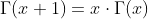Negative Values

Rearranging the above formular will give: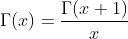The right side of this expression is defined for negative x in the range between -1 > x > 0.

Using the value -1/2 as value for x in this formular we get: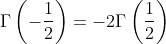For other negative values of x with x+n>0, except negative integers and zero (0, -1, -2, -3, ...) we can generalise the formular above: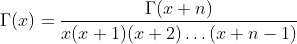Derivatives

First derivative of the gamma function: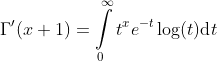N'th derivatives of the gamma function:Relation between the Riemann zeta function and the gamma function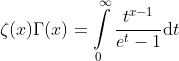Further Relations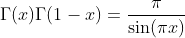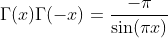Special Values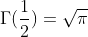Bild: "Edersee bei Niedrigwasser"

Next Page:

27. März 2021 Version 2.0
Copyright: Hermann Wacker Uhlandstraße 10 D-85386 Eching bei Freising Germany Haftungsausschluß